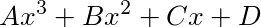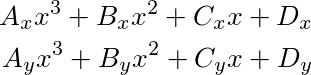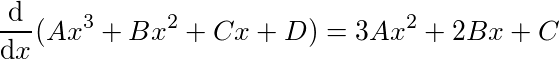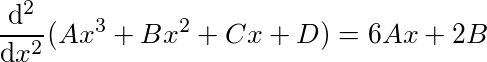# Making Splines Useful: Introduction

This post is the first in a 4 part series about using splines in real-time applications, such as computer games. Part 2: Closest Point, Part 3: Arc Length, and Part 4: Speed Control can be found at those links.

The emphasis of this discussion is on making splines useful, specifically useful for real-time applications, where update time is critical, and getting stuck in update loops is a big no-no. Splines are a tool that many developers are familiar with, but there is some quirkiness to dealing with splines in practice.

In this first article I will give a short introduction to splines and their usefulness for the wind currents in Seabird. I will also show how to get the slope and sharpness of the spline curve given any place on the spline. In part 2, I will show how to get the total length of the spline, and also how to find the closest point on the spline from any point in the world. In Part 3, I will show how to achieve a constant speed when moving along a spline path, given that you start with a spline where the control points are not at an equal distance from each other along the length of the spline.

To start off, a spline is defined by a set of control points. The placement of these control points give the shape of the spline. Between each consecutive set of control points there is a spline segment. Each segment is usually a cubic polynomial. The construction of a spline is such that all of the ends of these polynomials match up, and their first and second derivatives also match up, so that the spline can be treated like a single continuous parametric curve.If this is the first segment of the spline, then increasing x from 0 to 1 should interpolate smoothly from the first control point to the second control point. I have written A, B, C, and D as the coefficients for simplicity, but in the 2D case each of these coefficients have x and y components, so this is really 2 equations, one for each dimension:The equation for a higher dimensional spline segment is the same, given that there is an equation for each additional dimension.

In the cubic polynomial, D is the control point at the beginning of the segment. A, B, and C, are coefficients chosen such that when x is equal to the segment index we get D, the control point for the segment, and when x is equal to the segment index plus one, we get the control point at the beginning of the next segment in the spline. We then switch to using the polynomial of the next segment to smoothly interpolate to the third control point, and so on. The spline is also setup so that the first derivative and second derivative of the polynomials match up, so that moving along the spline path is smooth and continuous.

Splines are useful for the inner workings of the wind paths in my game Seabird. In Seabird, the characters are birds which are able to fly in wind currents that exist throughout the game. It is important that the movement of the characters through the wind currents appear smooth. I use splines to achieve this smooth movement.

One of the first tasks that you may want to do when working with splines is to find a vector facing down the spline from a given place on the spline. In Seabird, the speed the player is able to move faster down the wind current is proportional to how well their user input vector is aligned with the vector facing down the current. The vector facing down the current naturally is the current's slope or first derivative, which we can find by the power rule from calculus:If we use this equation in place of the original polynomial, then we get the slope at any given point on the spline. Notice how the D coefficient has disappeared. This is because the slope of the spline is not related the placement of the spline in the world. The slope of the spline should be the same no matter whether the spline is in China or Peru.

Another thing we might want to know is whether the spline is pinching tightly. This happens when the path has a sharp change in direction. We can find this by solving for the second derivative of the polynomial:We have seen the basic idea behind splines, and a few useful tools for knowing a little bit more about how the spline behaves. In the next article we will discuss how to get the total length of the spline. We will also discuss the problem of finding the closest point on the spline to any other point in the world. This can be useful in collision detection, because we need to know if an object is close enough to the spline to determine if it is overlapping.
Simon Anciaux,
I don't speak "math" very well so this article is a bit abstarct to me. Could you in the future provide code sample of how you use those formulas?

For example: 6Ax + 2B tells me if the spline is pinching tightly. But is it if the value is less then 0 it pinches and greater then 0 it doesn't ? Or closer to zero means pinching tightly ? If I got a code example it would be a lot easier to me.

And in the site's dark theme, your formulas are black on a dark background (the image background is transparent). It would probably be better to use a white background.
Timothy Barnes, Edited by Timothy Barnes on
That is a good question Simon.
By the way, I forgot this website has a black background mode. Thanks for letting me know about that.

Just like an object in a physics engine, splines have position, velocity, and acceleration. The second derivative of the spline segment is the spline’s acceleration. The acceleration of the spline behaves the same way as the acceleration vector you might have in a GameEntity structure.

For the second derivative, 6Ax + 2B, a value of 0 means that the spline is not twisting at the place x along the spline. If there is a region from A to B along the spline, and along this length the second derivative is 0, then A to B is straight. It is not curving in any direction.

If the second derivative is positive or negative, then that tells you how the spline is changing direction. Remember that A and B are vectors, so we actually have x and y components for each (in 2D). This means we have a x change and a y change. The length of this vector is useful for finding the total change. When a spline changes direction rapidly, this means the length of the second derivative vector will be large. I called this 'pinch', because a spline in this case tends to twist rapidly in a small area.

The spline segment code might be written like this:
 1 2 3 4 vec2 SplineSegment(vec2 A, vec2 B, vec2 C, vec2 D, float x) { return A*Power3(x) + B*Power2(x) + C*x + D; } 

Given a parameter, to find which segment you should be on is done like this:
 1 2 3 4 5 6 7 8 float splineParameter; int segmentIndex = Floor(splineParameter); float x = splineParameter - (float)segmentIndex; vec2 pointOnSpline = SplineSegment(coefficients[segmentIndex], coefficients[segmentIndex], coefficients[segmentIndex], coefficients[segmentIndex], x); 

To get the pinch of a place on the spline, just use the second derivative in place of the spline segment equation:
 1 2 3 4 vec2 SplineSegmentDxDx(vec2 A, vec2 B, float x) { return 6*A*x + 2*B; } 

 1 2 3 4 vec2 splineAcceleration = SplineSegmentDxDx(coefficients[segmentIndex], coefficients[segmentIndex], x); float splinePinch = Length(splineAcceleration); 

There are some useful videos about splines by Jorge Rodriguez. He also has source code on splines from a github page linked from his videos: